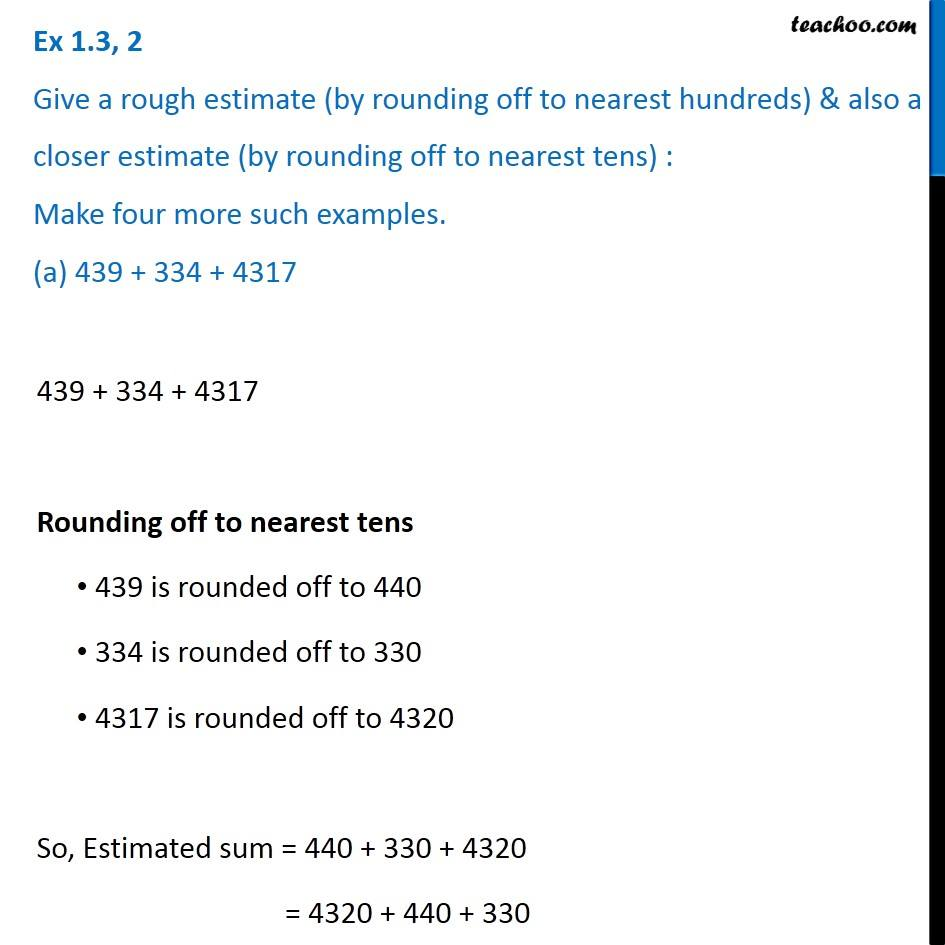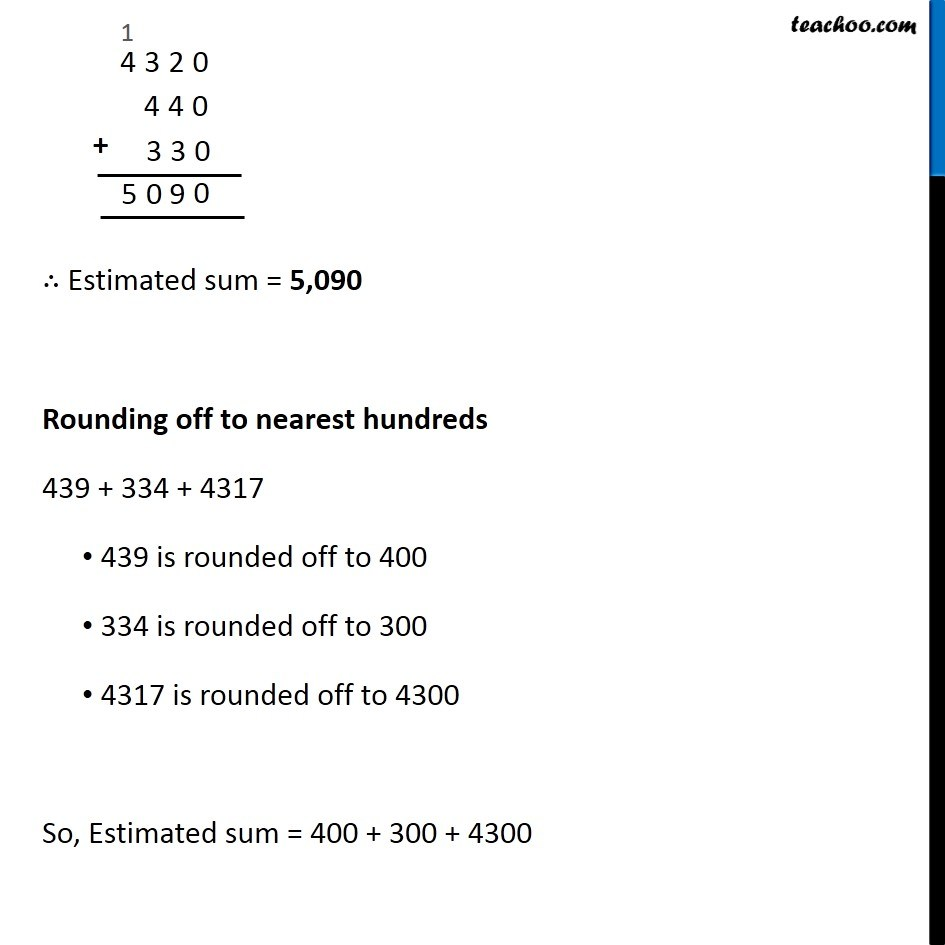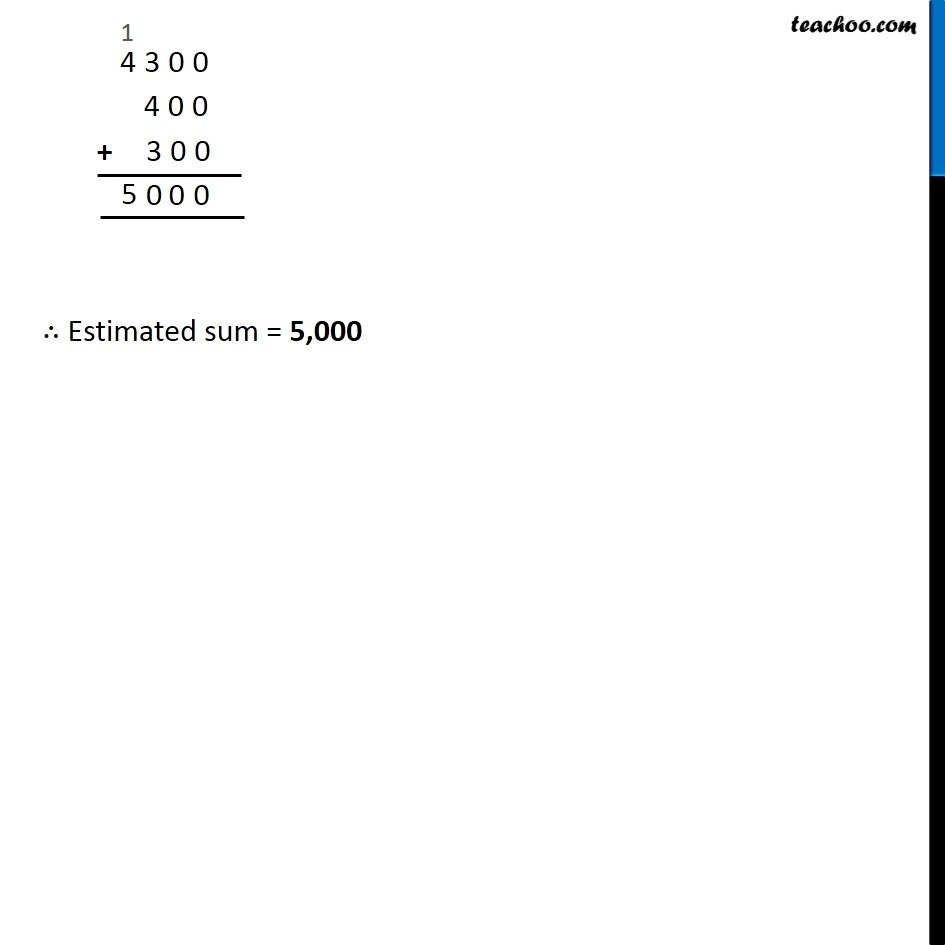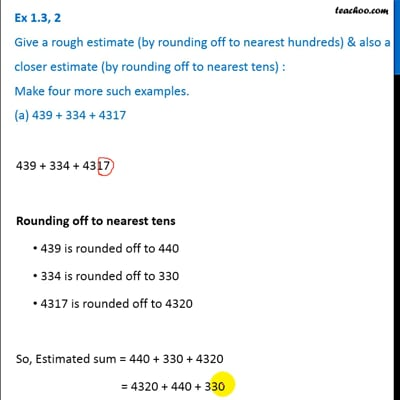Ex 1.3

Chapter 1 Class 6 Knowing our Numbers
Serial order wiseThis video is only available for Teachoo black users

### Transcript

Ex 1.3, 2 Give a rough estimate (by rounding off to nearest hundreds) & also a closer estimate (by rounding off to nearest tens) : Make four more such examples. (a) 439 + 334 + 4317439 + 334 + 4317 Rounding off to nearest tens 439 is rounded off to 440 334 is rounded off to 330 4317 is rounded off to 4320 So, Estimated sum = 440 + 330 + 4320 = 4320 + 440 + 330 ∴ Estimated sum = 5,090 Rounding off to nearest hundreds 439 is rounded off to 400 334 is rounded off to 300 4317 is rounded off to 4300 So, Estimated sum = 400 + 300 + 4300 ∴ Estimated sum = 5,000